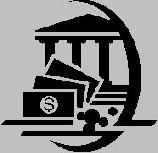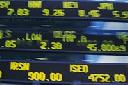Financial Terms Breakeven point

# Definition of Breakeven point## Breakeven point

The point at which total costs equal total revenue, i.e. where there is neither a profit nor a loss.

## breakeven point

The annual sales volume level at which total contribution
margin equals total annual fixed expenses. The breakeven point is only a
point of reference, not the goal of a business, of course. It is computed by
dividing total fixed expenses by unit margin. The breakeven point is
quite useful in analyzing profit behavior and operating leverage. Also, it
gives manager a good point of reference for setting sales goals and
understanding the consequences of incurring fixed costs for a period.

## Breakeven point

The sales level at which a company, division, or product line makes a
profit of exactly zero, and is computed by dividing all fixed costs by the average
gross margin percentage.

# Related Terms:

## Break-Even Analysis

An analytical technique for studying the relationships between fixed cost, variable cost, and profits. A breakeven chart graphically depicts the nature of breakeven analysis. The breakeven point represents the volume of sales at which total costs equal total revenues (that is, profits equal zero).

## contribution margin

An intermediate measure of profit equal to sales revenue
minus cost-of-goods-sold expense and minus variable operating
expenses—but before fixed operating expenses are deducted. Profit at
this point contributes toward covering fixed operating expenses and
toward interest and income tax expenses. The breakeven point is the
sales volume at which contribution margin just equals total fixed
expenses.

## fixed expenses (costs)

Expenses or costs that remain the same in amount,
or fixed, over the short run and do not vary with changes in sales volume
or sales revenue or other measures of business activity. Over the
longer run, however, these costs increase or decrease as the business
grows or declines. Fixed operating costs provide capacity to carry on
operations and make sales. Fixed manufacturing overhead costs provide
production capacity. Fixed expenses are a key pivot point for the analysis
of profit behavior, especially for determining the breakeven point and for
analyzing strategies to improve profit performance.

## margin of safety

the excess of the budgeted or actual sales
of a company over its breakeven point; it can be calculated
in units or dollars or as a percentage; it is equal to
(1 - degree of operating leverage)

## Basis point

In the bond market, the smallest measure used for quoting yields is a basis point. Each percentage
point of yield in bonds equals 100 basis points. Basis points also are used for interest rates. An interest rate of
5% is 50 basis points greater than an interest rate of 4.5%.## Basis Point

One one-hundredth of one percent

## Basis point

One hundredth of one percentage point, or 0.0001.

## Basis Point

One one-hundredth of a percentage point, used to express variations in yields. For example, the difference between 5.36 percent and 5.38 percent is 2 basis points.

## Bond points

A conventional unit of measure for bond prices set at \$10 and equivalent to 1% of the \$100 face
value of the bond. A price of 80 means that the bond is selling at 80% of its face, or par value.

## break-even point (BEP)

the level of activity, in units or dollars, at which total revenues equal total costs

## Cash-flow break-even point

The point below which the firm will need either to obtain additional financing
or to liquidate some of its assets to meet its fixed costs.

## Delivery points

Those points designated by futures exchanges at which the financial instrument or
commodity covered by a futures contract may be delivered in fulfillment of such contract.

## Free-on-Board (FOB) Shipping Point

A shipping arrangement agreed to between buyer and
seller where title to the goods sold passes when the goods in question are delivered to a common
carrier. When goods are shipped FOB shipping point, revenue is properly recognized when the
goods are delivered to the common carrier.

## Order penetration point

The point in the production process when a product is
reserved for a specific customer.## order point

the level of inventory that triggers the placement
of an order for additional units; it is determined based
on usage, lead time, and safety stock

## Outbound stock point

A designated inventory location on the shop floor between
operations where inventory is stockpiled until needed by the next operation.

## Point

The smallest unit of price change quoted or, one one-hundredth of a percent. Related: minimum price
fluctuation and tick.

## Point and figure chart

A price-only chart that takes into account only whole integer changes in price, i.e., a
2-point change. point and figure charting disregards the element of time and is solely used to record changes
in price.

## Point and figure chart

A financial chart usually used to plot asset price data.
Upward price movements are plotted as X’s and downward price movements
are plotted as O’s.

## point of sale (POS)

The terminal at which a customer uses his/her debit card to make a direct payment transaction. See also Interac Direct Payment.

## Point-of-use delivery

A delivery of stock to a location in or near the shop floor
adjacent to its area of use.

## Point-of-use storage

The storage of stock in a location in or near the shop floor
adjacent to its area of use.

## Price value of a basis point (PVBP)

Also called the dollar value of a basis point, a measure of the change in
the price of the bond if the required yield changes by one basis point.

## split-off point

the point at which the outputs of a joint process are first identifiable or can be separated as individual products

## Split-off point

The point in a production process when clearly identifiable joint costs
can be identified within the process.

## Stockpoint

An inventory storage area used for short-term inventory staging.

## Turning Point

The trough or peak of a business cycle.